﻿ 一文搞懂JavaScript中原型与原型链 - 自由资讯

# 一文搞懂JavaScript中原型与原型链

##### 目录
• 1.构造函数原型prototype
• 2.对象原型__proto__
• 3.constructor构造函数
• 4.原型链
• 5.原型对象中的this指向
• 6.扩展内置对象（原型对象的应用）

```function student(uname,age) {
this.uname = uname;
this.age = age;
this.school = function() {
console.log('深圳理工大学');
}
}
var stu1 = new student('小红',18);
var stu2 = new student('小紫',20);```

## 1.构造函数原型prototype

JavaScript规定,每一个构造函数都有一个prototype属性, 指向另一个对象。注意这个prototype就是一个对象,这个对象的所有属性和方法,都会被构造函数所拥有。

```function student(uname,age) {
this.uname = uname;
this.age = age;
}
student.prototype.school = function() {
console.log('深圳理工大学');
}
var stu1 = new student('小红',18);
var stu2 = new student('小紫',20);```

## 2.对象原型__proto__

`console.log(stu1.__proto__ === student.prototype);`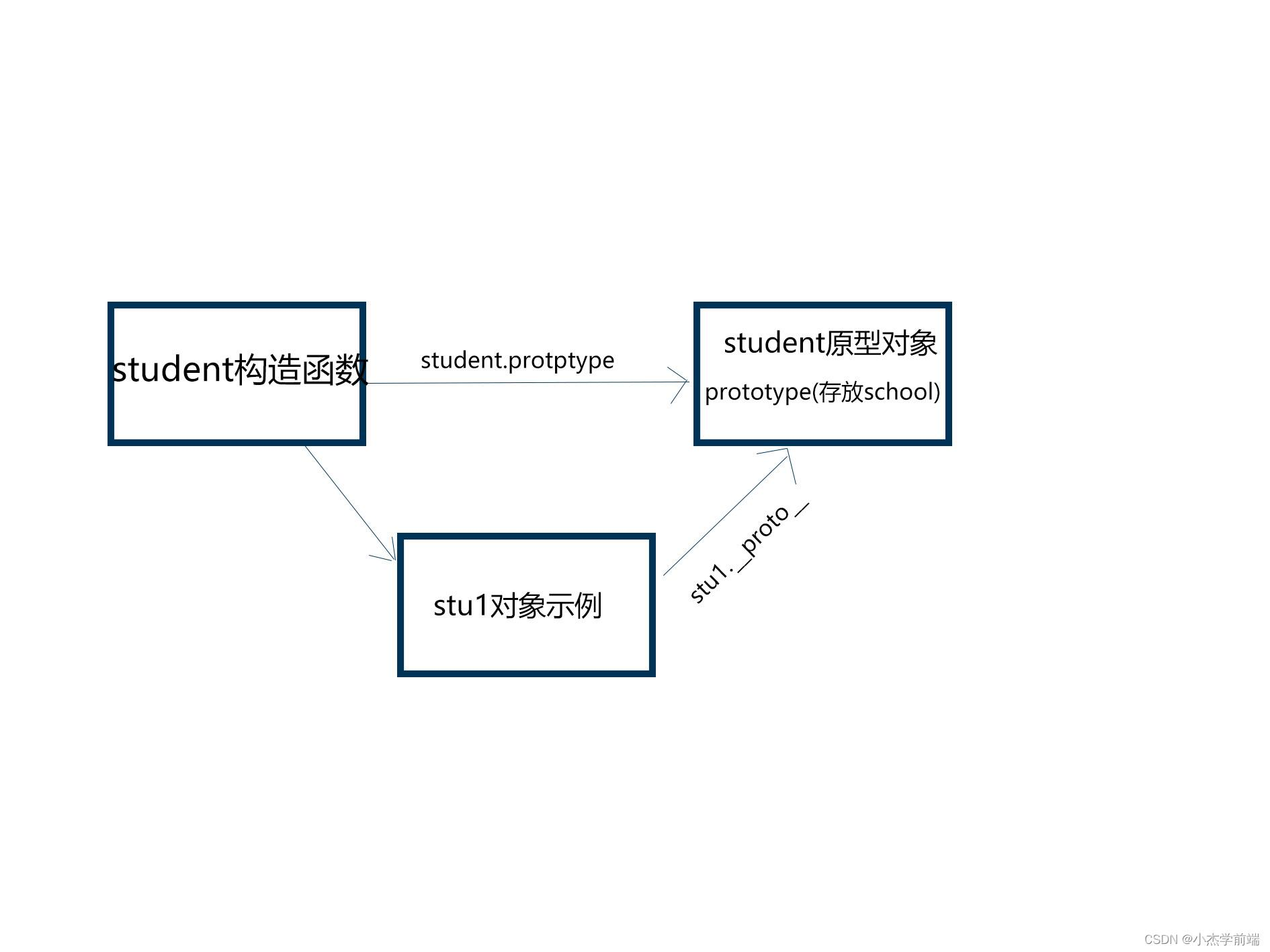## 3.constructor构造函数

```console.log(student.prototype);
console.log(stu1.__proto__);```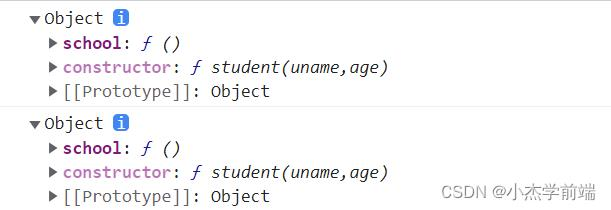constructor主要用于记录该对象引用于哪个构造函数，它可以让原型对象重新指向原来的构造函数

```student.prototype = {
school:function() {},
location:function() {}
}```

```console.log(student.prototype.constructor);
console.log(stu1.__proto__.constructor);```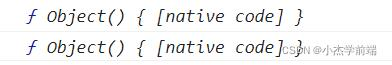```student.prototype = {
constructor:student,
school:function() {},
location:function() {}
}```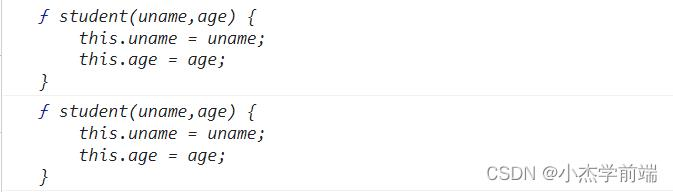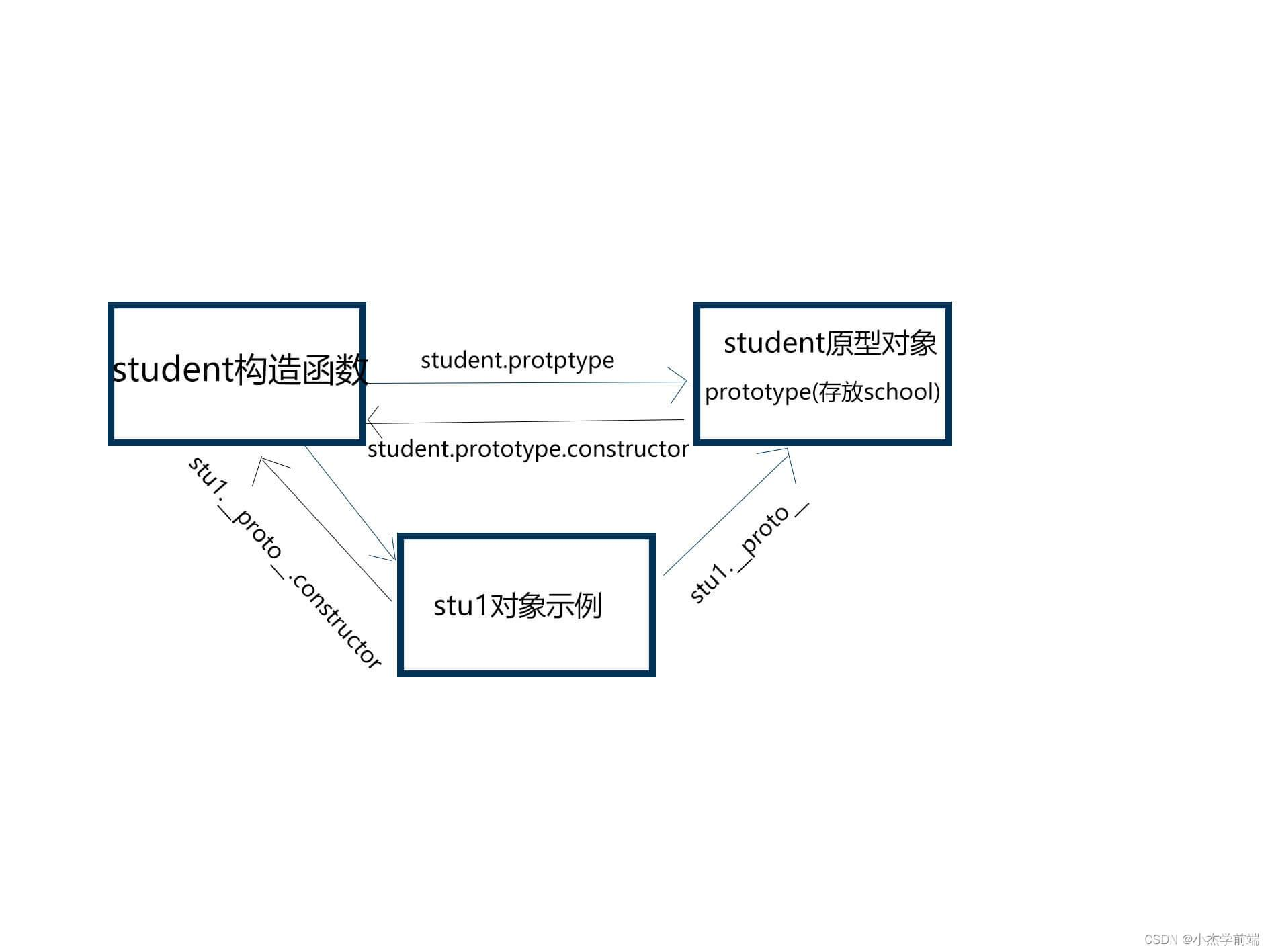## 4.原型链

```function student(uname,age) {
this.uname = uname;
this.age = age;
}
student.prototype.school = function() {
console.log('深圳理工大学');
}
var stu1 = new student('小红',18);
console.log(student.prototype);```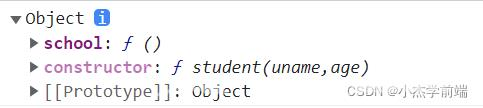`console.log(student.prototype.__proto__);`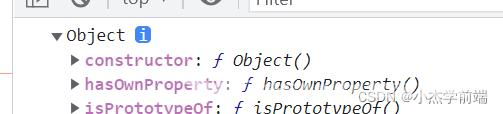Object原型对象是由谁创建出来的呢，毫无疑问是Object构造函数创建出来的。那么我们继续，Object原型对象也是一个对象，那它也有一个原型，这个原型指向的又是谁呢？

`console.log(Object.prototype.__proto__);`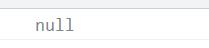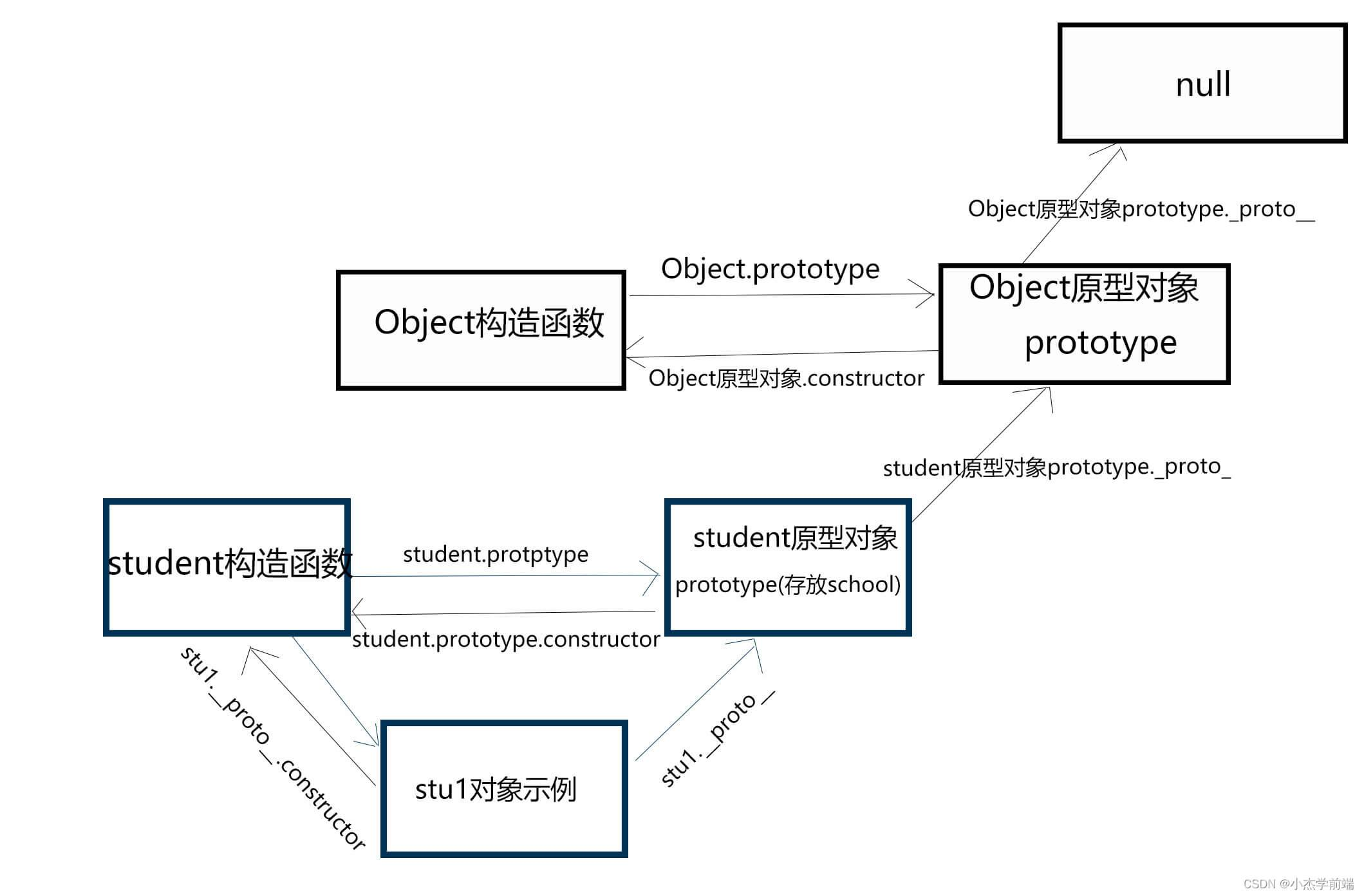## 5.原型对象中的this指向

```function student(uname,age) {
this.uname = uname;
this.age = age;
}
var that;
student.prototype.school = function() {
that = this;
console.log('深圳理工大学');
}
var stu1 = new student('小红',18);
stu1.school();
console.log(that === stu1);```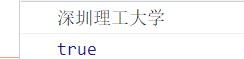## 6.扩展内置对象（原型对象的应用）

`console.log(Array.prototype);`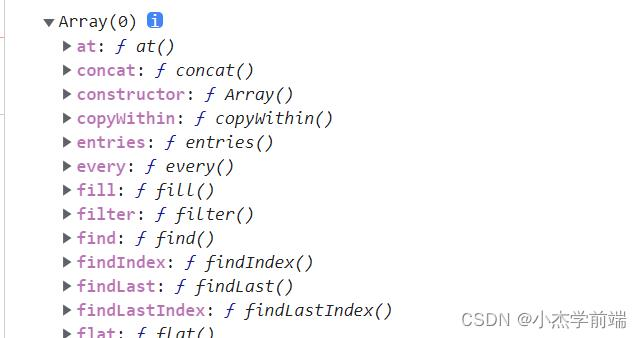```Array.prototype.sum = function() {
var sum = 0;
for(var i = 0;i<this.length;i++)
{
sum += this[i];
}
return sum;
}
var ss = new Array(4,5,3,6);
console.log(ss.sum());```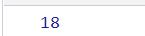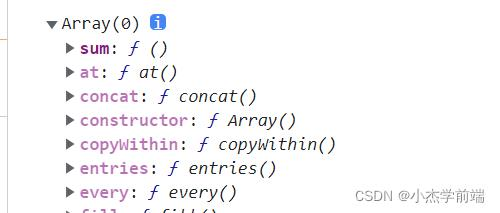﻿